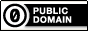Recommended Posts

Hello guys,

I need a little help for make a multidimensional array in this way:

I have a string delimited with "|" and i need to split between column, i have already make it:

abc abc abc

Now from every colum i need to split each character in this way

a a a

b b b

c c c

But if the character is between [ ] leave it in a single row, like:

a        a  a

b        b  [a12]

c        c   c

[a33]

d

f

Is clear enought? If not i'll try to explain better. My initial script:

#include <Array.au3> ; Only for _ArrayDisplay()

\$sString = "testabc[12a]|test2589|test3578[34asd]|test4877"
StringReplace(\$sString, "|", "|")
\$iCol = @extended

Global \$aArray[\$iCol+1]
\$aCols = StringSplit(\$sString, "|", 1)
For \$c = 1 To \$aCols
\$aArray[\$c - 1] = \$aCols[\$c]
Next

_ArrayDisplay(\$aArray, "\$aArray")
; until here is ok

For \$x = 0 To UBound(\$aArray, 2) - 1
;StringSplit(\$aArray[\$x], "", 1) ; split each char
Next

Thanks

Edited by johnmcloud

Share on other sites

Johnmcloud,

Like this???

#include <Array.au3> ; Only for _ArrayDisplay()

\$sString = "testabc[12a]|test2589|test3578[34asd]|test4877|abcxyz"
StringReplace(\$sString, "|", "|")
\$iCol = @extended

Global \$aArray[\$iCol+1]
\$aCols = StringSplit(\$sString, "|", 1)
For \$c = 1 To \$aCols
\$aArray[\$c - 1] = \$aCols[\$c]
Next

;_ArrayDisplay(\$aArray, "\$aArray")
; until here is ok

local \$space = true, \$sTmp
For \$x = 0 To UBound(\$aArray, 2) - 1
for \$1 = 1 to stringlen(\$aArray[\$x])
if stringmid(\$aArray[\$x],\$1,1) = '[' then \$space = not \$space
if not \$space and stringmid(\$aArray[\$x],\$1,1) = ']' then \$space = not \$space
\$sTmp &= (\$space) ? stringmid(\$aArray[\$x],\$1,1) & ' ' : stringmid(\$aArray[\$x],\$1,1)
Next
\$aArray[\$x] = \$sTmp
\$sTmp = ''
Next

_ArrayDisplay(\$aArray)

It's 4:00AM and I could NOT work out the regexp for this so you get it the long winded way...

edit: corrected the for loop (should have started at 1

Edited by kylomas

Forum Rules         Procedure for posting code

"I like pigs.  Dogs look up to us.  Cats look down on us.  Pigs treat us as equals."

- Sir Winston Churchill

Share on other sites

NoLike this:

#include <Array.au3> ; Only for _ArrayDisplay()

\$sString = "testabc[12a]|test2589|test3578[34asd]|abcxyzuea"

Local \$aArray
\$aArray = "t"
\$aArray = "e"
\$aArray = "s"
\$aArray = "t"
\$aArray = "a"
\$aArray = "b"
\$aArray = "c"
\$aArray = "[12a]"

\$aArray = "t"
\$aArray = "e"
\$aArray = "s"
\$aArray = "t"
\$aArray = "2"
\$aArray = "5"
\$aArray = "8"
\$aArray = "9"

\$aArray = "t"
\$aArray = "e"
\$aArray = "s"
\$aArray = "t"
\$aArray = "3"
\$aArray = "5"
\$aArray = "7"
\$aArray = "8"
\$aArray = "[34asd]"

\$aArray = "a"
\$aArray = "b"
\$aArray = "c"
\$aArray = ""
\$aArray = "x"
\$aArray = "y"
\$aArray = "z"
\$aArray = "u"
\$aArray = "e"
\$aArray = "a"
_ArrayDisplay(\$aArray)

Thanks anyway

Share on other sites

johnmcloud,

This seems to work:#include <Array.au3> ; Only for _ArrayDisplay()

\$sString = "testabc[12a]|test2589|test3578[34asd]|abcxyzuea"

Local \$aArray
\$aArray = "t"
\$aArray = "e"
\$aArray = "s"
\$aArray = "t"
\$aArray = "a"
\$aArray = "b"
\$aArray = "c"
\$aArray = "[12a]"

\$aArray = "t"
\$aArray = "e"
\$aArray = "s"
\$aArray = "t"
\$aArray = "2"
\$aArray = "5"
\$aArray = "8"
\$aArray = "9"

\$aArray = "t"
\$aArray = "e"
\$aArray = "s"
\$aArray = "t"
\$aArray = "3"
\$aArray = "5"
\$aArray = "7"
\$aArray = "8"
\$aArray = "[34asd]"

\$aArray = "a"
\$aArray = "b"
\$aArray = "c"
\$aArray = ""
\$aArray = "x"
\$aArray = "y"
\$aArray = "z"
\$aArray = "u"
\$aArray = "e"
\$aArray = "a"
_ArrayDisplay(\$aArray, "Original", Default, 8)

; ###############################

; Split string on delimiter
\$aSplit_1 = StringSplit(\$sString, "|")

; Create array with correct number of columns
Global \$aFinalArray[\$aSplit_1]

; Loop through the split array
For \$i = 1 To \$aSplit_1

; Extract line
\$sLine = \$aSplit_1[\$i]
; Get line length
\$iLen = StringLen(\$sLine)
; Create hold all chars if necessary
Global \$aSplit_2[\$iLen + 1]
; Set indices
\$iWriteindex = 0
For \$iReadIndex = 1 To \$iLen
; Get char
\$sChar = StringMid(\$sLine, \$iReadIndex, 1)
; Add to array
\$aSplit_2[\$iWriteindex] = \$sChar
; if starting a [...] section
If \$sChar = "[" Then
Do
; Keep looping through the line
\$sChar = StringMid(\$sLine, \$iReadIndex, 1)
; Add all chars to the same element until end of [...] section
\$aSplit_2[\$iWriteindex] &= \$sChar
Until \$sChar = "]"
EndIf
; Move to next element
\$iWriteindex += 1
Next

; Just to see what happened
;_ArrayDisplay(\$aSplit_2, \$sLine, Default, 8)

; ReDim final array if required
If UBound(\$aFinalArray) < \$iWriteindex Then
ReDim \$aFinalArray[\$iWriteindex][\$aSplit_1]
EndIf

; Add elements to final array
For \$j = 0 To \$iWriteindex - 1
\$aFinalArray[\$j][\$i - 1] = \$aSplit_2[\$j]
Next

Next

; And here is the result
_ArrayDisplay(\$aFinalArray, "M23", Default, 8)
M23Any of my own code posted anywhere on the forum is available for use by others without any restriction of any kind

Open spoiler to see my UDFs:

Spoiler

ArrayMultiColSort ---- Sort arrays on multiple columns
ChooseFileFolder ---- Single and multiple selections from specified path treeview listing
Date_Time_Convert -- Easily convert date/time formats, including the language used
ExtMsgBox --------- A highly customisable replacement for MsgBox
GUIExtender -------- Extend and retract multiple sections within a GUI
GUIFrame ---------- Subdivide GUIs into many adjustable frames
GUIListViewEx ------- Insert, delete, move, drag, sort, edit and colour ListView items
GUITreeViewEx ------ Check/clear parent and child checkboxes in a TreeView
Marquee ----------- Scrolling tickertape GUIs
NoFocusLines ------- Remove the dotted focus lines from buttons, sliders, radios and checkboxes
Notify ------------- Small notifications on the edge of the display
Scrollbars ----------Automatically sized scrollbars with a single command
StringSize ---------- Automatically size controls to fit text
Toast -------------- Small GUIs which pop out of the notification area

Share on other sites

Yes, seems work fineThanks Melba, i'll check it out

EDIT: Checked and integrated with the main script, work without problems. Thanks again

Edited by johnmcloud

Create an account or sign in to comment

You need to be a member in order to leave a comment

Create an account

Sign up for a new account in our community. It's easy!

Register a new account